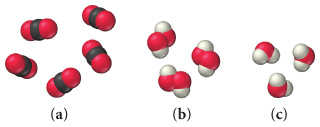# Problem: Write a ratio showing the relationship between the amounts of each element for each of the following:Part (a):A) 3O:C B) 2O:C C) O:C D) O:2C

###### FREE Expert Solution

O → red sphere

C → black sphere

90% (288 ratings)###### Problem Details

Write a ratio showing the relationship between the amounts of each element for each of the following:Part (a):

A) 3O:C

B) 2O:C

C) O:C

D) O:2C

Frequently Asked Questions

What scientific concept do you need to know in order to solve this problem?

Our tutors have indicated that to solve this problem you will need to apply the Law of Definite Proportions concept. You can view video lessons to learn Law of Definite Proportions. Or if you need more Law of Definite Proportions practice, you can also practice Law of Definite Proportions practice problems.

What professor is this problem relevant for?

Based on our data, we think this problem is relevant for Professor Walker's class at HFCC.